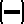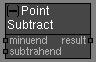# Point Subtract node

Left Toolbar >Math Nodes >Point Subtract

The Point Subtract node allows you to execute a subtraction operation between two node Point values.

## Procedure

### To execute a point subtraction operation in the node graph:

1. On the left toolbar choose Point Nodes> Point Subtract2. Drag the Point Subtract icon into the Node Graph.3. Connect the point Nodes to be subtracter to the minuend and subtrahend inputs.

4. Connect the Output: result .

## Inputs

Minuend First point from which the second point is to be subtracted. A POINT type of value.

Subtrahend Second point, is the number to be subtracted from minuend. A POINT type of value.

## Outputs

result

A POINT type of value.(x,y)

## Attributes PanelPoint

Minuend. This attribute is exposed as input by default. Right-click the Node to hide this attribute as input and set the point values manually.

Subtrahend. This attribute is exposed as input by default. Right-click the Node to hide this attribute as input and set the point values manually.

Math Nodes

Main Page